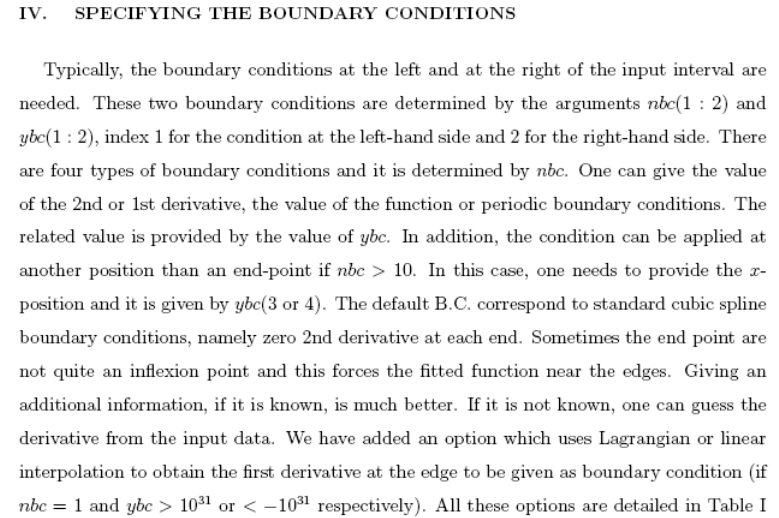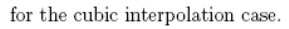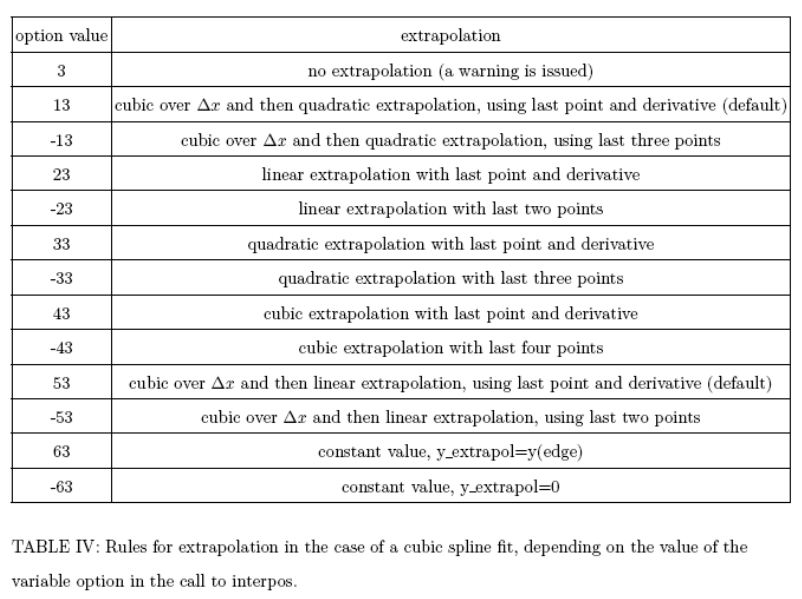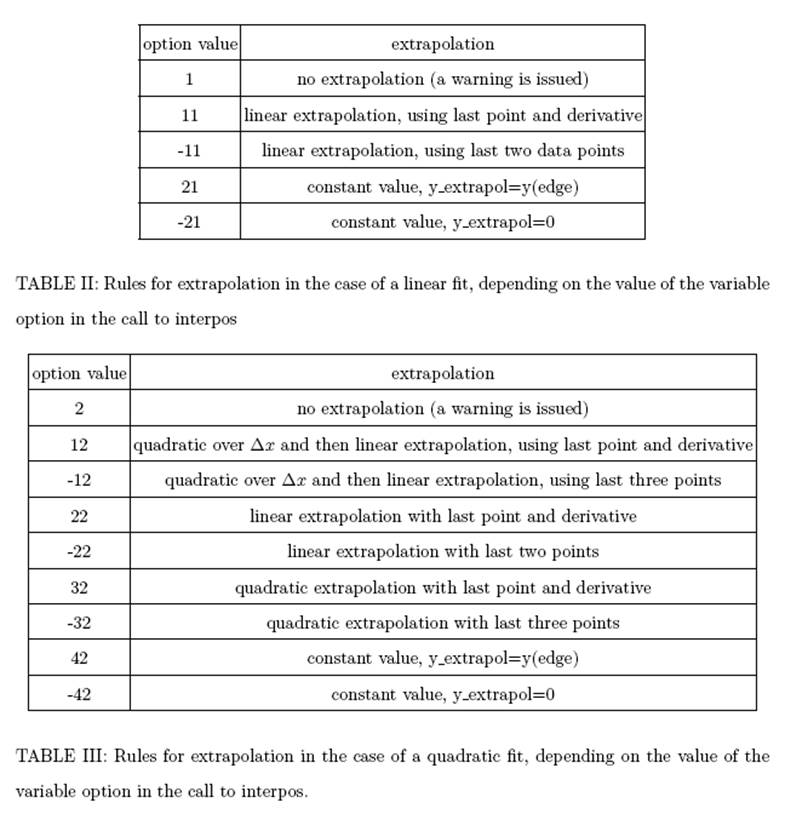INTERPOS module and routines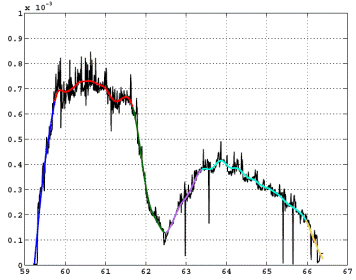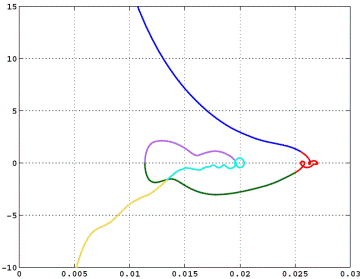INTERPOS is a set of f90 routines which allows very easily and efficiently to interpolate data, to compute 1st or second derivative, to compute integral of a function or to extrapolate. It has also a very efficient matlab interface (see below), python, idl as well

You can see some examples on how to install the various libraries in the file INSTALL

It can also smooth the data to the desired level with a"tension", using cubic splines with tension which minimizes the second derivatives.

INTERPOS is open access and you can use the code at your wish, but we ask you to fill in the following agreement: INTERPOS_docx (pdf) and send it toYou can get the last version from SPC-EPFL gitlab server:

- once you have sent the signed agreement you can perform for example:

git clone https://gitlab.epfl.ch/spc/interpos.git interpos

(you can ask to be registered as a member to use git clone git@gitlab.epfl.ch:spc/interpos.git  )

(Note that the svn server is obsolete, now we use git)

INTERPOS can do (see below for extrapolation options for the various cases):

- Linear interpolation/extrapolation

- Cubic spline interpolation/extrapolation (standard or with smoothing)

For the cubic spline fit (standard or with tension) one can define various boundary conditions:

- 2nd derivative

- 1st derivative

- Value of function at given point

- Periodic boundary conditions

Some examples of calls:

USE interpos_module

Call interpos(xin,yin,nin,xout=x,yout=y)               ! computes y, cubic spline with 2nd der.=0 at end points on x=x

Call interpos(xin,yin,nin,xout=x,yout=y,youtp=yp,youtpp=ypp,youtint=yint)                ! Also computes dy/dx, d2y/dx and int(y dx)

With default smoothing

Call interpos(xin,yin,nin,nout,tension=-1.,x,y,yp,ypp,yint)

With 1st derivative=0 at left and 2nd=0 at right:

Call interpos(xin,yin,nin,nout,tension=-1.,x,y,yp,ypp,yint,nbc=(/1,0/),ybc=(/0.,0./))

With periodic boundary conditions:

Call interpos(xin,yin,nin,nout,tension=-1.,x,y,yp,ypp,yint,nbc=-1,ybc=2.*pi)

To compute first the spline coefficients (the 2nd derivative) and then use it for many interpolations:

call interpos(xin,yin,yinnew,yinpp,nin,[tension,nbc,ybc,sigma])                 ! only computes yinpp

call interpos(xin,yinnew,yinpp,nin,nout,xout,yout,youtp,youtpp,youtint,option])    ! only computes yout's

The full interface:

Vector xout:

SUBROUTINE interpos(XIN,YIN,NIN,NOUT,tension,xout,yout,youtp,youtpp,youtint,nbc,ybc,sigma,option,info)

Scalar xout:

SUBROUTINE interpos(X,Y,N,xscal,tension,yscal,yscalp,yscalpp,yscalint,nbcscal,ybcscal,sigma,option,info)

Matlab interface:

A mex file is available (make interpos_for_matlab) in the matlab subdirectory. It can be compiled within matlab with:

>> mex interpos.f90

Examples are available in the matlab and matlab/example directories, but typically:

>> [yfit,dyfit,d2yfit,yint]=interpos(xin,yin,xout,-1.); % uses interpos with default tension to compute function, 1st, 2nd derivatives and integral on xout.

The use of interpos is faster than the spline routine in matlab, so it can be used with tension=0. on large arrays efficiently.

Matlab quick install:

For the matlab interface you need the interpos.m file and the mex file in your ~/matla directory:

Linux 32 bits: interpos.mexglx

Linux 64 bits: interpos.mexa64

Full files, fortran and matlab:

Last release: tag 9.0.2

From version 8.3 Python (thanks to Antoine Merle) and idl interfaces have been added

contact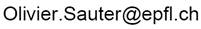for further information

More details: